# Ace

The length of segment AB is 24 cm, and the point M and N divided it into thirds. Calculate the circumference and area of this shape.

o =  75.3982 cm
S =  150.7964 cm2

### Step-by-step explanation: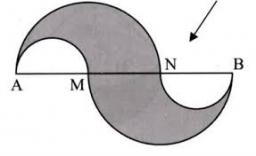Did you find an error or inaccuracy? Feel free to write us. Thank you!Tips to related online calculators
Do you want to convert length units?

#### You need to know the following knowledge to solve this word math problem:

We encourage you to watch this tutorial video on this math problem:

## Related math problems and questions:

• Rectangle diagonalsIt is given a rectangle with an area of 24 cm2 a circumference of 20 cm. The length of one side is 2 cm larger than the length of the second side. Calculate the length of the diagonal. Length and width are yet expressed in natural numbers.
• 2d shapeCalculate the content of a shape in which an arbitrary point is not more than 3 cm from the segment AB. The length of the segment AB is 5 cm.
• Segment in a triangle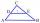In a triangle ABC with the side/AB/ = 24 cm is constructed middle segment/DE/ = 18 cm parallel to the side AB at a distance 1 cm from AB. Calculate the height of the triangle ABC to side AB.
• Circle and hexagon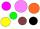Calculate the radius of a circle whose circumference is 8.4 cm longer than the inscribed regular hexagon's circumference.
• RhombusIt is given a rhombus of side length a = 19 cm. Touchpoints of inscribed circle divided his sides into sections a1 = 5 cm and a2 = 14 cm. Calculate the radius r of the circle and the length of the diagonals of the rhombus.
• Tangent 3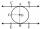In a circle with centre O radius is 4√5 cm. EC is the tangent to the circle at point D. Segment AB IS THE DIAMETER of given circle. POINT A is joined with POINT E and POINT B is joined with POINT C. Find DC if BC IS 8cm.
• ChordIt is given to a circle k(r=6 cm) and the points A, B such that / AB / = 8 cm lies on k. Calculate the distance of the center of circle S to the midpoint C of the segment AB.
• RhumblineFind circumference and area of the rhumbline ABCD if the short side AD of which has a length of 5 cm, and the heel of the height from D leading to the AB side divides the AB side into two sections of 3 cm and 4 cm.
• Trapezoid thirdsThe ABCD trapezoid with the parallel sides of the AB and the CD and the E point of the AB side. The segment DE divides the trapezoid into two parts with the same area. Find the length of the AE line segment.
• Parallelogram ABCDWe have the parallelogram ABCD, where AB is 6.2 cm BC is 5.4 cm AC is 4.8 cm calculate the height on the AB side and the angle DAB
• Four circles1) Calculate the circle radius if its area is 400 cm square 2) Calculate the radius of the circle whose circumference is 400 cm. 3) Calculate circle circumference if its area is 400 cm square 4) Calculate the circle's area if perimeter 400 cm.
• Rectangular trapezoidThe rectangular trapezoid ABCD is: /AB/ = /BC/ = /AC/. The length of the median is 6 cm. Calculate the circumference and area of a trapezoid.
• Trapezoid - intersection of diagonalsIn the ABCD trapezoid is AB = 8 cm long, trapezium height 6 cm, and distance of diagonals intersection from AB is 4 cm. Calculate the trapezoid area.
• Chord circleThe circle to the (S, r = 8 cm) are different points A, B connected segment /AB/ = 12 cm. AB mark the middle of S'. Calculate |SS'|. Make the sketch.
• The regularThe regular triangular prism has a base in the shape of an isosceles triangle with a base of 86 mm and 6.4 cm arms, the height of the prism is 24 cm. Calculate its volume.
• Chord 4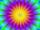I need to calculate the circumference of a circle, I know the chord length c=22 cm and the distance from the center d=29 cm chord to the circle.
• Two chordsCalculate the length of chord AB and perpendicular chord BC to circle if AB is 4 cm from the center of the circle and BC 8 cm from the center of the circle.